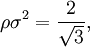Difference between revisions of "Building up a triangular lattice"$\rho\sigma^2=\frac{2}{\sqrt{3}},$
where$\sigma$ is the distance between nodes of the lattice. The dual of this lattice is the honeycomb lattice.